# Bounding the chromatic number of graphs with no odd hole (Solved)

 Importance: High ✭✭✭
 Author(s): Gyarfas, Andras
 Subject: Graph Theory » Coloring » » Vertex coloring
 Keywords: chi-bounded coloring induced subgraph odd hole perfect graph
 Posted by: mdevos on: June 25th, 2007
 Solved by: Alex Scott and Paul Seymour http://arxiv.org/abs/1410.4118
Conjecture   There exists a fixed functionso thatfor every graphwith no odd hole.

For a graph, we let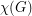denote the chromatic number, andthe size of the largest clique. A hole is an induced cycle of length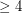, so an odd hole is an induced cycle with length an odd number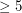.

Thanks to the perfect graph theorem, we know that a graph is perfect (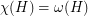for all induced subgraphs) if and only if it has no odd hole, and no complement of an odd hole. Perfect graphs are a particularly pretty and well-behaved class, and the proof of the perfect graph theorem gives us a great deal of structural information about them. On the other hand, graphs with no odd hole are considerably more wild, so it appears unlikely that this conjecture could be established by way of a precise structure theorem.

The conjecture is trivial in the case when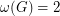, as in this casehas no odd cycle, so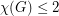. The special case when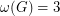was resolved by Robertson, Seymour, and Thomas who found a structural description of these graphs which implies that they are 4-colorable.

Gyarfas also posed a related conjecture that the same conclusion should hold true for the family of graphs which do not contain sufficiently large holes (more precisely, for everythere is a functionso that every graphwith no hole of size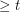satisfies). Graphs with no hole of sizeare chordal and have clique number equal to their chromatic number, so they satisfy this conjecture. I (M. DeVos) believe it is open in all other cases.

## Bibliography

*[G] A. Gyarfas, Problems from the world surrounding perfect graphs, [in Proceedings of the International Conference on Combinatorial Analysis and its Applications (Pokrzywna, 1985)]. Zastos. Mat. 19 (1987), no. 3-4, 413--441 MathSciNet

* indicates original appearance(s) of problem.